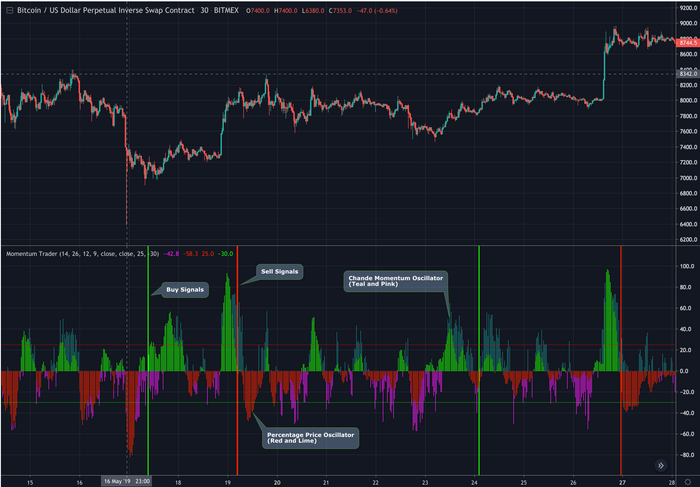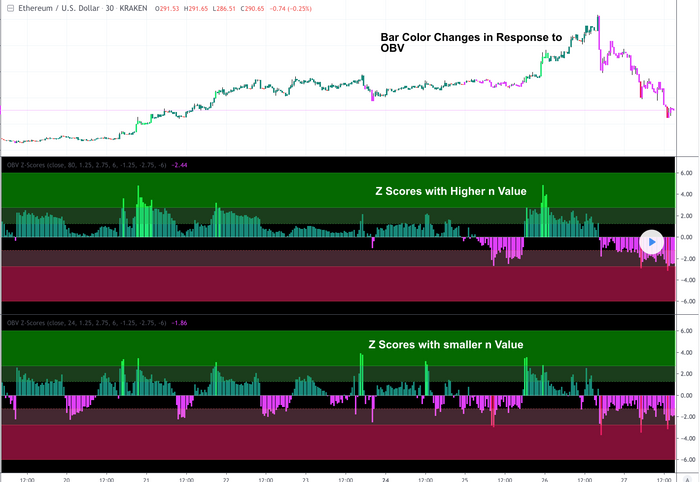Home / News / Tagged: BITCOin

# Blog - News 

Filter by tag:

Posts tagged "BITCOin"

# New Momentum Trader- Trading ViewClick Here to View on Trading View

This study combines two versatile momentum indicators :
Chande Momentum Oscillator:
-Measures trend strength, with higher absolute values meaning greater strength.
-Also tracks divergence. When price increases, but is not accompanied by an increase in Chande Momentum Oscillator values, it signifies bearish divergence and a reversal is likely to follow.
-Shown as the teal and pink histogram.

Percentage Price Oscillator:
-Similar to the MACD , except that it expresses the difference between the two moving averages in terms of a percentage. This makes it a little easier to visualize.
-PPO values greater than zero indicate an uptrend, as that means the fast EMA is greater than the slow (and vice versa).

Entry and Exit Conditions:
Enter When:
1) Chande Momentum crosses over zero from negative to positive territory. AND
2) It has been less than 3 bars since Chande Momentum was less than the lower green line. AND
3) Chande Momentum is rising(positive slope).

Exit When:
1) Chande Momentum is greater than the upper line. AND
2) It has been less than 6 bars since the PPO value was greater than the upper bound. AND
3) PPO is less than 5 (meaning the difference between the two EMA's is less than 5%). AND
4) PPO has a negative slope.

This study comes with alert conditions for long entries and exits.

~Happy Trading~

# ETH/USD OBV Z-Scores New Scripthttps://www.tradingview.com/i/6ZBAadtS/

This study calculates the On-Balance Volume (OBV) and displays it in terms of its Z-Score.

OBV is a great momentum indicator . As the name suggests, OBV predicts changes in price based on the security's volume flow.

Formula:
if (Current Price > Previous Price)
then Current OBV = Previous OBV + Current Volume

if (Current Price < Previous Price)
then Current OBV = Previous OBV - Current Volume

if (Current Price == Previous Price)
then Current OBV = Previous OBV

As the formula shows, the OBV goes a step beyond just looking at the pure volume of a security. Instead, it factors in relative price action from period to period to reflect investor sentiment. As a result, we often look to the OBV to spot bullish or bearish trends while they are in the early stages of development or simply predict impending uptrends or downtrends.

To make the OBV easier to visualize, we converted the value to a Z-Score. The Z-Score is a simple statistical measurement and represents the current OBV value's distance from the mean OBV value in terms of # of standard deviations.

Users can adjust the values for the 2 upper bounds for Z Scores and 2 lower bounds. Additionally, the n value for z score calculation can be adjusted in the input menu. A higher n value means the z score will be based on a longer lookback period. A lower value will result in more sensitive readings.

Overall, I think this is an interesting way to represent OBV values and will be a valuable leading indicator.

~Happy Trading~